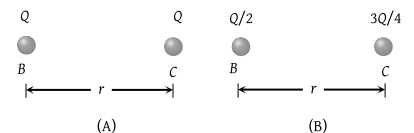NEET Questions Solved

Two spherical conductors B and C having equal radii and carrying equal charges in them repel each other with a force F when kept apart at some distance. A third spherical conductor having same radius as that of B but uncharged is brought in contact with B, then brought in contact with C and finally removed away from both. The new force of repulsion between B and C is

(1) F/4

(2) 3 F/4

(3) F/8

(4) 3 F/8

(4) Initially $F=k.\frac{{Q}^{2}}{{r}^{2}}$ (fig. A). Finally when a third spherical conductor comes in contact alternately with B and C then removed, so charges on B and C are Q / 2 and 3Q / 4 respectively (fig. B)Now force $F\text{'}=k.\frac{\left(\frac{Q}{2}\right)\text{\hspace{0.17em}}\left(\frac{3Q}{4}\right)}{{r}^{2}}=\frac{3}{8}F$

Difficulty Level:

• 21%
• 19%
• 14%
• 48%